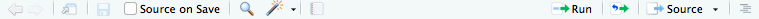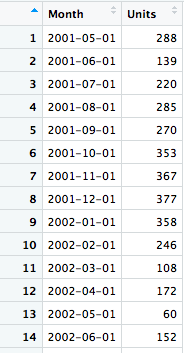top of page# How to decompose a time series using R

### Prerequisites

This article assumes no knowledge of R. Hence a very detailed step by step procedure is given.

Time series analysis is very important for business who operate in the inventory based business or service business like transportation, call centres etc.

It is very important to predict the future demand as understocking the inventory will lead to loss of business opportunity and overstocking or creating unnecessary capacity will lock up the funds which would have been used for any other purpose.

Either case scenario is not good for the business.

## Before we start making a prediction

But before we start making predictions there is a lot of work to do. Check following time series.This is a sales data of a company for particular products. It is not an actual data but a simulated one. As you can see there are a lot of variations in this series. Prima facie this is a very random series.

Let’s look at the series again and we will observe that

1. There is a certain pattern that is repeating. We can see that the sale is going up and then down and then this cycle is repeating itself. This is the seasonality that is present in the series.

2. Next, we can see that overall the sales figure is growing with time. This means there is a trend in this time series.

3. The series is growing and there is some seasonality but there are some flat portions in between and there are some abnormally low values and high values. So there must be some remainder or an error that is not explained by either trend or seasonality. So this error becomes the third part of our time series.

These are the three portions that make up our series.

Effectively we can write

$Y=S+T+e$

This model is called an additive model.

Not necessarily these parts always add up. Sometimes they multiply each other. In that case, we can write

$Y=S\times T\times e$

This model is called a multiplicative model.

However, we can convert a multiplicative model into an additive model just by taking a log of both sides of a multiplicative model. As you know log function converts arithmetic multiplication into an addition.

$\log_{}{Y}=\log_{}{S}+ \log_{}{T}+ \log_{}{e}$

Here we are assuming the base of the log to be ‘e’. But you can take any base suitable for your application.

## So how we are going to decompose a time series?

Are we going to decompose a time series using manual calculation? No! absolutely not. We will be doing it through R language.

R is an open source statistical language which will make your life very easy with the statistical analysis.

If you do not have R installed on your computer then you can install it from here. Select the appropriate operating system and you are good to go. For actually writing R scripts, I use RStudio. You can download the RStudio from here.

RStudio is compatible with Linux, Windows as well as MacOS.

Once you are ready with RStudio, follow these steps to decompose a time series into different components.

### 1.

Create a new project in RStudio by clicking on File -> New Project -> New Project (if you do not have a project directory if you have a project directory then click on Existing Project.)### 2.

After the project is created, copy the CSV file in the project directory you are using. Download the dataset used for this article using the following form. (Dataset no longer available)

### 3.

Open the dataset in Excel or any spreadsheet viewer or CSV viewer to check the file. This will give you an overview of the columns used in the dataset and how the data is structured.You can see that the first column contain dates in the format dd/mm/yy. There is a difference of a month in each preceding row. That means this data is monthly. You encounter datasets that may have weekly, daily, quarterly, even hourly, minute wise or second wise frequency. But in this case, we are dealing with months only.

The next column is sales in the number of units. Actually, just looking at the dataset we cannot ascertain that the sales figure is in the number of units and not in monetary terms. That’s why usually you get a data dictionary with each dataset. Data dictionary gives the description of each column and the permissible values etc.

### 4.

Create a new R script in RStudio by clicking on the File and then New File and click on R Script. Save this file.

### 5.

Now we will start analysing the dataset. To do this we have to load this dataset in R. R provides a data structure called Data Frame. A dataframe is like a spreadsheet with rows and columns.

Load the data in the dataframe using following code. You can replace ‘df’ with any other name. Type the name of the file in double quotes.

df <- read.csv("ts.csv")



### 6.

After writing the above code, click on the Source button. This will run the code.### 7.

After clicking source we will see how the dataframe actually looks. To do this click on the table icon on the far left of the ‘df’. Check following image for a guideline.Here is how your dataframe should look.### 7.

As you can see in our CSV file the name of one of the column was Month but here in dataframe it is appearing to be ‘X…Month’. Many times what happens, you have column names that are not easy to understand. Hence it is always a good idea to rename the columns in such a case. In this step, we will rename the columns. Type this command and then press source.

When you are just starting with R programming, it is a good practice to type one line of code or a block of code and press source. This will give you an idea of what each line or block of code is for.

colnames(df) <- c("Month", "Units")



Here c(“Month”, “Units”) is called a vector. You can add multiple values to an ‘R’ vector. In short, a vector is a collection of different elements.

When you have all string or characters in an ‘R’ vector, then it is a character vector. If you have all numbers in a vector, then it is a numeric vector. When you have both strings and numbers in a vector then the numbers will be automatically converted into the strings. And hence the vector is of character type.

Once you execute this code, check out the dataframe again. You will see changed column names.

### 8.

When you are dealing with time series, it is important to have a column that contains date or time values. Here in our dataframe, we have a column named ‘Month’. However, you are able to read the date, not necessarily, R can read it as a date. Hence we need to convert the ‘Month’ column to R readable date.

We can access a column of a dataframe with the name of the dataframe, followed by that followed by name of the column. So, if we want to access the column, ‘Month’ column of dataframe ‘df’, we would write ‘df$Month’ Write the following code to convert the column to a proper format. df$Month <- as.Date(df$Month, format="%d/%m/%y")  Execute the code by clicking Source. Here we are converting the Month column of the dataframe and again storing it back to the same column. But if you want to store it in some different vector then you could type z <- as.Date(df$Month, format="%d/%m/%y")



Here we are storing the converted column in the vector ‘z’. Do not type this line in our code. This is just for your reference.

In the above code, you can see that, as.Date function has two values separated by a comma. The first value to the left of the comma is the vector or a column of the dataframe that you want to convert. And second value after the comma is the format of date present in the unconverted or original column. We have a date in our dataframe stored as dd/mm/yy

Refer to the following table. And accordingly, type the format. Let’s say you have the data stored as 17-Jan-2017. Then the format that you will use will be “%d-%b-%Y”SymbolMeaningExample%dDay as a number01-31%aAbbreviated weekdayMon%AUnabbreviated weekdayMonday%mMonth00-12%bAbbreviated monthJan%BUnabbreviated monthJanuary%y2 digits year18%Y4 digits year2018

After you convert the column, again check the dataframe. The values that you are seeing in the Month column are now ‘R’ readable dates.### 9.

By now, the data preparation part is over. Let’s plot our time series now. R has a builtin function to draw plots. Type the following code and press Source.

plot(x=df$Month, y=df$Units, type='l')



Here we are giving x parameter, y parameter and type as ‘l’. If we omit type, then a scatter plot will be plotted.

Once you run this code by clicking source, you will see the following plot.### 10.

Although plotting this time series does not contribute to the overall output of the program. But as a statistician or data analyst, you should plot various plots. Plots can give you various insights.

### 11.

Till now, we were dealing with the dataframe. But R provides another data type called time series (ts). So, we will convert the Units column to a time series. R provides a builtin function ‘ts’ which converts data to a time series. Type the following code and press Source.

units <- ts(df$Units, frequency = 12)  Here we are passing two values to the ‘ts’ function. First one is the Units column from the dataframe ‘df’ and second is the frequency of the time series. In our case, we have monthly data. Hence we are giving a frequency of 12. We are storing this time series in the variable ‘units’ ### 11. Here we go, we have reached the final step. R provides another builtin function to decompose a time series called ‘stl’. STL stands for Seasonal Decomposition of Time Series by Loess. Execute following code to decompose our time series. decomp <- stl(units, s.window = "periodic")  We are giving two values to the ‘stl’ function. First one is an actual time series, which we had stored in the ‘units’ variable. And second value which asks R to extract periodic seasonality. We are storing the result of this function in another variable ‘decomp’. Click Source after typing this line. So we have a decomposed time series. But now we have to actually see the decomposed parts. To do this, type following code and click Source. plot(decomp)  You should get the following outputThat’s it! We have decomposed a time series into different parts. Here is the complete code for your reference df <- read.csv("ts.csv") colnames(df) <- c("Month", "Units") df$Month <- as.Date(df$Month, format="%d/%m/%y") plot(x=df$Month, df$Units, type='l') units = ts(df$Units, frequency = 12)

decomp <- stl(units, s.window = "periodic")

plot(decomp)



What we have seen here is a very basic time series decomposition. Because, sometimes, some data is missing, there are some text values when there should be numbers, sometimes the data is incorrectly entered or there are duplicate entries. So at times, the data preparation part is quite exhaustive than the one we encountered for this example.

This is how you decompose a time series.

If you have any question regarding time series or above code then just enter it in the chat box.

## Note:

I am no more writing regarding Python or programming on this blog, as I have shifted my focus from programming to WordPress and web development. If you are interested in WordPress, you can continue reading other articles on this blog. Thanks and Cheers ????